Next: Eigenstates of and Up: Spin Angular Momentum Previous: Spin Operators

# Spin Space

We now have to discuss the wavefunctions upon which the previously introduced spin operators act. Unlike regular wavefunctions, spin wavefunctions do not exist in real space. Likewise, the spin angular momentum operators cannot be represented as differential operators in real space. Instead, we need to think of spin wavefunctions as existing in an abstract (complex) vector space. The different members of this space correspond to the different internal configurations of the particle under investigation. Note that only the directions of our vectors have any physical significance (just as only the shape of a regular wavefunction has any physical significance). Thus, if the vectorcorresponds to a particular internal state then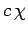corresponds to the same state, whereis a complex number. Now, we expect the internal states of our particle to be superposable, since the superposability of states is one of the fundamental assumptions of quantum mechanics. It follows that the vectors making up our vector space must also be superposable. Thus, if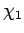and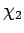are two vectors corresponding to two different internal states then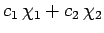is another vector corresponding to the state obtained by superposing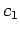times state 1 withtimes state 2 (whereandare complex numbers). Finally, the dimensionality of our vector space is simply the number of linearly independent vectors required to span it (i.e., the number of linearly independent internal states of the particle under investigation).

We now need to define the length of our vectors. We can do this by introducing a second, or dual, vector space whose elements are in one to one correspondence with the elements of our first space. Let the element of the second space which corresponds to the elementof the first space be called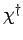. Moreover, the element of the second space which corresponds tois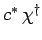. We shall assume that it is possible to combineandin a multiplicative fashion to generate a real positive-definite number which we interpret as the length, or norm, of. Let us denote this number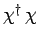. Thus, we have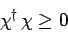(713)

for all. We shall also assume that it is possible to combine unlike states in an analogous multiplicative fashion to produce complex numbers. The product of two unlike statesand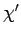is denoted. Two statesandare said to be mutually orthogonal, or independent, if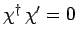.

Now, when a general spin operator,, operates on a general spin-state,, it coverts it into a different spin-state which we shall denote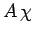. The dual of this state is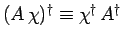, where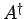is the Hermitian conjugate of(this is the definition of an Hermitian conjugate in spin space). An eigenstate ofcorresponding to the eigenvaluesatisfies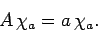(714)

As before, ifcorresponds to a physical variable then a measurement ofwill result in one of its eigenvalues (see Sect. 4.10). In order to ensure that these eigenvalues are all real,must be Hermitian: i.e.,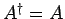(see Sect. 4.9). We expect the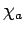to be mutually orthogonal. We can also normalize them such that they all have unit length. In other words,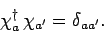(715)

Finally, a general spin state can be written as a superposition of the normalized eigenstates of: i.e.,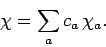(716)

A measurement ofwill then yield the resultwith probability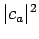.Next: Eigenstates of and Up: Spin Angular Momentum Previous: Spin Operators
Richard Fitzpatrick 2010-07-20